Home MonkeyNotes Printable Notes Digital Library Study Guides Message Boards Study Smart Parents Tips College Planning Test Prep Fun Zone Help / FAQ How to Cite New Title Request

 5.7 Properties of Similar triangles Perimeters of similar triangles: Perimeters of similar triangles are in the same ratio as their corresponding sides and this ratio is called the scale factor. In figure 5.6 there are two similar triangles . D LMN and D PQR.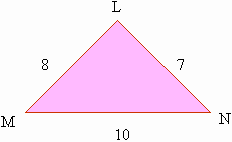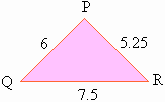Figure 5.6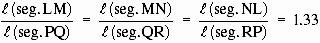This ratio is called the scale factor. Perimeter of D LMN = 8 + 7 + 10 = 25 Perimeter of D PQR = 6 + 5.25 + 7.5 = 18.75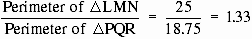Thus, the perimeters of two similar triangles are in the ratio of their scale factor. Areas of similar triangles: The ratio of the areas of two similar triangles is equal to the ratio of the squares of the corresponding sides, i.e. the square of the scale factor.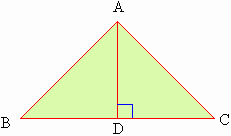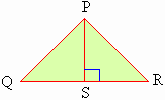Figure 5.7 D ABC ~ D PQR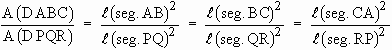To prove that Draw perpendicular from A and P to meet seg.BC and seg.QR at D and S respectively. Since D ABC ~ D PQR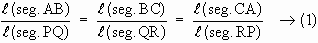also Ð B @ Ð Q In D ABD and D PQS also Ð B @ Ð Q and Ð ADB @ Ð PSQ \ D ABD ~ D PQS by A A test.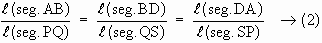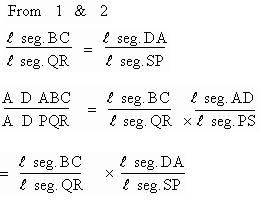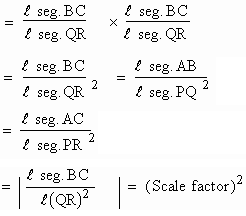Thus the areas of two similar triangles are in the same ratio as the square of their scale factors. Index 5.1 Introduction 5.2 Ratio And Proportionality 5.3 Similar Polygons 5.4 Basic Proportionality Theorem 5.5 Angle Bisector Theorem 5.6 Similar Triangles 5.7 Properties Of Similar Triangles
 Search: All Products Books Popular Music Classical Music Video DVD Toys & Games Electronics Software Tools & Hardware Outdoor Living Kitchen & Housewares Camera & Photo Cell Phones Keywords: